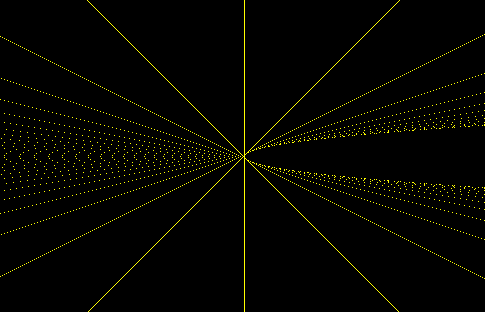# Visualizing Galois groups of quadratics

Yesterday Jack Kennedy told me about a graph he’d made as part of a project he’s working on and I asked if I could post it here.

The Galois group of a quadratic polynomial x2 + bx + c is either A2 or S2. If b2 – 4c is a perfect square, the polynomial has rational roots and the Galois group is the trivial group A2. Otherwise there are distinct irrational roots and the Galois group is the two-element group S2.

As b and c range over integers, color a pixel yellow if the group is A2 and black otherwise. This produces the image below.Note that what appear to be the crossed lines y = ±x intersecting at 0 are actually the lines y = ±(x+1) intersecting at (-1,0).

You can find a larger image here. View the page source to see the JavaScript that produced the image. The page is calculating and setting the value of one million pixels, and yet the time to render the page isn’t even noticeable.